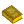## Complexity Theory

The theory of classifying problems based on how difficult they are to solve. A problem is assigned to the P-Problem (Polynomial time) class if the number of steps needed to solve it is bounded by some Power of the problem's size. A problem is assigned to the NP-Problem (nondeterministic Polynomial time) class if it permits a nondeterministic solution and the number of steps of the solution is bounded by some power of the problem's size. The class of P-Problems is a subset of the class of NP-Problems, but there also exist problems which are not NP.

However, if a solution is known to an NP-Problem, it can be reduced to a single period verification. A problem is NP-Complete if an Algorithm for solving it can be translated into one for solving any other NP-Problem. Examples of NP-Complete Problems include the Hamiltonian Cycle and Traveling Salesman Problems. Linear Programming, thought to be an NP-Problem, was shown to actually be a P-Problem by L. Khachian in 1979. It is not known if all apparently NP-Problems are actually P-Problems.

ReferencesComputational Complexity

Bridges, D. S. Computability. New York: Springer-Verlag, 1994.

Brookshear, J. G. Theory of Computation: Formal Languages, Automata, and Complexity. Redwood City, CA: Benjamin/Cummings, 1989.

Cooper, S. B.; Slaman, T. A.; and Wainer, S. S. (Eds.). Computability, Enumerability, Unsolvability: Directions in Recursion Theory. New York: Cambridge University Press, 1996.

Garey, M. R. and Johnson, D. S. Computers and Intractability: A Guide to the Theory of NP-Completeness. New York: W. H. Freeman, 1983.

Goetz, P. ``Phil Goetz's Complexity Dictionary.'' http://www.cs.buffalo.edu/~goetz/dict.html.

Hopcroft, J. E. and Ullman, J. D. Introduction to Automated Theory, Languages, and Computation. Reading, MA: Addison-Wesley, 1979.

Lewis, H. R. and Papadimitriou, C. H. Elements of the Theory of Computation, 2nd ed. Englewood Cliffs, NJ: Prentice-Hall, 1997.

Sudkamp, T. A. Language and Machines: An Introduction to the Theory of Computer Science, 2nd ed. Reading, MA: Addison-Wesley, 1996.

Welsh, D. J. A. Complexity: Knots, Colourings and Counting. New York: Cambridge University Press, 1993.